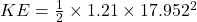## show answer No Attempt 50% Part (b) Now consider a disk of mass 8 kg with radius 0.55 m. Disk rotates around itself once every 0.35 sec. Wha

Question

show answer No Attempt 50% Part (b) Now consider a disk of mass 8 kg with radius 0.55 m. Disk rotates around itself once every 0.35 sec. What is the rotational energy of this disk?

in progress 0
4 weeks 2021-08-19T13:28:22+00:00 2 Answers 0 views 0

Explanation:

mass of disc, m = 8 kg

radius of disc, r = 0.55 m

Time period, t = 0.35 s

Angular velocity, ω = 2π/T

ω = 2 x 3.14 / 0.35 = 17.94 rad/s

moment of inertia of the disc, I = 0.5 mr²

I = 0.5 x 8 x 0.55 x 0.55 = 1.21 kgm²

The rotational kinetic energy is given by

K = 0.5 x I x ω²

K = 0.5 x 1.21 x 17.94 x 17.94 = 194.72 J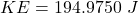Explanation:

Given:

mass of disk,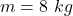radius of the disk,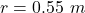time taken by the disk to complete on rotation,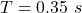We know that rotational kinetic energy is given as: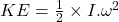……………………….(1)

Now the angular speed of the disk: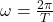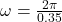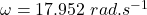The moment of inertia of a disk is given as: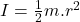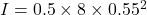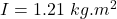Now using eq. (1):